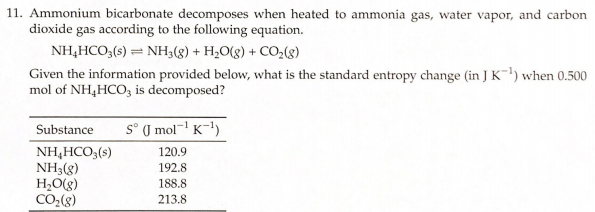# 11. Ammonium bicarbonate decomposes when heated to ammonia gas, water vapor, and carbon dioxide gas according to the following equation. NH4HCO3(s) ⇌ NH3(g) + H2O(g) + CO2(g) Given the information provided below, what is the standard entropy change (in J K^-1) when 0.500 mol of NH4HCO3 is decomposed?